# How to declare and Initialize two dimensional Array in Java with Example

An array of more than one dimension is known as multi-dimensional array. Two of the most common examples of multi-dimensional arrays are two and three dimensional array, known as 2D and 3D array, anything above is rare. I have never seen 4 dimensional arrays, even 3D arrays are not that common. Now question comes, when do use multi-dimensional array? Any real life example? Well, 2D arrays are very common on platform games like Super Mario Bros to represent screen or terrain; 2D arrays can also be used to represent structures like spreadsheet, or to draw board games like Chess, which requires 8x8 board, Checkers and  Tic-Tac-Toe, which requires 3 rows and 3 columns. Another popular application of multi-dimensional arrays are in matrix manipulation. For example to represent a 3x3 matrix you need a two dimensional array of 3 one dimensional array each containing 3 elements.

Similarly to represent 3x2 matrices you need 2 two dimensional array of one dimensional array of length 3. In other words, each row in two dimensional array is a one dimensional array.  Java truly doesn't support multi-dimensional array but allows you to create and use array of any number of dimensional.

Two dimensional array is actually an array of one dimensional array. This is unlike languages like C or FORTRAN, which allows Java array to have rows of varying length i.e. a multidimensional array can have 2 columns in one row and 3 columns in second.

Similar to one dimensional array, length of two dimensional array is also fixed. You can not change length of any array, i.e. number of rows and columns will remain fixed. A 2x2 array can hold total 4 elements and they can be accessed using row and column index e.g. a will give you elements in first row and first column, similarly a will give you elements from 2nd row and 2nd column. Just like normal array, index start at 0 and finishes at length -1.

## How to Declare 2 Dimensional Array in Java

If you know how to create one dimensional array and fact that multi-dimensional arrays are just array of array in Java, then creating a 2 dimensional array is very easy. Instead of one bracket, you will use two e.g. int[][] is a two dimensional integer array. You can define a 2D array in Java as follows :
```int[][] multiples = new int;     // 2D integer array with 4 rows and 2 columns
String[][] cities = new String;  // 2D String array with 3 rows and 3 columns```
By the way when you initially declare a two dimensional array, you must remember to specify first dimension, for example following array declaration is illegal in Java.
``` int[][] wrong = new int[][]; // not OK, you must specify 1st dimension
int[][] right = new int[]; // OK```
The first expression will throw "Variable must provide either dimension expressions or an array initializer" error at compile time. On the other hand second dimension is optional and even if you don't specify compiler will not complain, as shown below :
```String[][] myArray = new String[]; // OK
String[][] yourArray = new String; // OK```
This is possible because a two dimensional array in Java is nothing but an array of one dimensional array, because of this, you can also create a two dimensional array where individual one dimensional arrays has different length, as seen in following example.
```class TwoDimensionalArray {

public static void main(String[] args) {
String[][] salutation = {
{"Mr. ", "Mrs. ", "Ms. "},
{"Kumar"}
};

// Mr. Kumar
System.out.println(salutation + salutation);

// Mrs. Kumar
System.out.println(salutation + salutation);
}
}

The output from this program is:

Mr. Kumar
Mrs. Kumar```
In this example, you can see that salutation is a 2D array but its first row has 3 elements while second row has just one element.

You can access elements of a two dimensional array either by using both indexes or just one index. For example salutation represent a Single String in Java, while salutation  represent a one dimensional array ( a single row in the 2 dimensional array).

## How to Initialize Two Dimensional Array in Java

So far we have just declared and created the array, we haven't initialized them. Which means all elements of array has their default values e.g. zero for array of integral values e.g. byte, short, char and int,  0.0 for floating point array e.g. float and double, false for boolean arrays and null for array of reference type e.g. String array elements. You can verify this by accessing first element of two dimensional array as multiples, which will print zero, as shown below.
```           boolean[][] booleans = new boolean;
System.out.println("booleans : " + booleans);

byte[][] bytes = new byte;
System.out.println("bytes : " + bytes);

char[][] chars = new char;
System.out.println("chars : " +  (int)chars);

short[][] shorts = new short;
System.out.println("short : " + shorts);

int[][] ints = new int;
System.out.println("ints : " + ints);

long[][] longs = new long;
System.out.println("longs : " + longs);

float[][] floats = new float;
System.out.println("floats : " + floats);

double[][] doubles = new double;
System.out.println("doubles : " + doubles);

Object[][] objects = new Object;
System.out.println("objects : " + objects);

Output
booleans : false
bytes : 0
chars : 0
short : 0
ints : 0
longs : 0
floats : 0.0
doubles : 0.0
objects : null```
You can see default values of different types of primitive arrays here. Character array is bit tricky because if you print 0 as character it will print a null character and that's why I have used it's integer value by casting to int.

Now there are two ways to initialize a two dimensional array in Java, either by using array literal at the time of creation or by using nested for loop and going through each element. In next example we will learn how to loop through a two dimensional array, initialize each element and how to print a two dimensional array in Java.
```       // initializing two dimensional array as literal
String[][] names = {
{"Sam", "Smith"},
{"Robert", "Delgro"},
{"James", "Gosling"},
};

// how to initialize two dimensional array in Java
// using for loop
int[][] board = new int;

for (int i = 0; i < board.length; i++) {
for (int j = 0; j < board[i].length; j++) {
board[i][j] = i + j;
}
}```
In first example, we have created and initialized String array using array literal, while in second example we have created a two dimensional array board, and later initialized it by looping through the array.

## How to Loop and Print 2D array in Java

If you want to access each element of a two dimensional array, then you need to iterate through two dimensional array using two for loops. Why? because you need two indexes to access individual element from 2D array. You can either use advanced for each loop or classic for loop with counter. Second one is more powerful as it provides explicit counter which can be used as indexes. In order to print contents of a two dimensional array, you can either use this method or can use Arrays.deepToString() method, which will return a String version of all elements of a 2D array, as shown in following example.
```import java.util.Arrays;

/**
* Java Program to initialize and print two dimensional array in Java.
*
* @author WINDOWS 8
*
*/
class Basics {

public static void main(String args[]) {

// initializing two dimensional array as literal
String[][] names = {
{"John", "Smith"},
{"Javin", "Paul"},
{"James", "Gosling"},
};

// how to initialize two dimensional array in Java
// using for loop
int[][] board = new int;

for (int i = 0; i < board.length; i++) {
for (int j = 0; j < board[i].length; j++) {
board[i][j] = i + j;
}
}

// now let's print a two dimensional array in Java
for (int[] a : board) {
for (int i : a) {
System.out.print(i + "\t");
}
System.out.println("\n");
}

// printing 2D array using Arrays.deepToString() method
System.out.println("another way to print 2D arrays");
System.out.println(Arrays.deepToString(board));

}

}
Output:
0   1   2

1   2   3

2   3   4

another way to print 2D arrays
[[0, 1, 2], [1, 2, 3], [2, 3, 4]]```

to learn more about printing array elements, see how to print array in Java

## Important Points about Multi-dimensional Array in Java

1)  Java doesn't support multi-dimensional array in true sense. In a true two dimensional array all the elements of array occupy a contiguous block of memory , but that's not true in Java. Instead a multi-dimensional array is an array of array. For example two dimensional array in Java is simply an array of one dimensional array like String[][] is an array of array of String[] or "array of array of strings".  This diagram shows how exactly two dimensional arrays are stored in Java :

2) Because of above reason, second dimension in array is optional in Java. You can create a two dimensional array without specifying both dimension e.g. int[] is valid array declaration. It also allows you  create multi-dimensional array whose rows can vary in length, as we have seen in our second example.

3) While creating two dimensional or three dimensional array in Java, first dimension is must, without that compile will throw error e.g. int[] is Not Ok but int[] is Ok.

4) Two dimensional array is very useful data structure in game programming. You can use it on tile based game e.g. Super Mario Bros to draw terrain, background and other object, on games like Tetris to represent playing area. 2D array is also very useful in matrix manipulation. You can use 2D array to represent any matrix and perform addition, multiplication and other operations. 2D array can also be used to represent any object in plain using X and Y coordinate. Similarly 3D arrays can be used to represent things in three dimensional space using X, Y and Z coordinates. Some of the popular example of two dimensional arrays are chess board, checkers board and other board games which has slots. You can view chess board as an array of 8 rows and 8 columns.

5) There are multiple ways to define and initialize multidimensional array in Java, you can either initialise them using in the line of declaration or some time later using a nested for loop. You will need as many for loop as many dimension of array you have. For example to explicitly initialize a three dimensional array you will need three nested for loops. On other hand, to initialize a 2D array, you just need two nested for loops.

6) In a two dimensional array like int[][] numbers = new int, there are three rows and two columns. You can also visualize it like 3 integer array of length 2. You can find number of rows using numbers.length and number of columns using numbers.length expression, as shown in below example. This is also very useful while iterating over two dimensional array in Java.
```int[][] primes = new int;

int rows = primes.length; // 3
int cols = primes.length; // 2

System.out.printf("int has %s rows and %d columns %n", rows, cols);

Output : int has 3 rows and 2 columns```

That's all about multi-dimensional array in Java. It is one of the useful data structure, especially to represent two dimensional things e.g. matrix. It is also very useful to build tile based games. By the way,  It's worth to remember that Java doesn't support true multi-dimensional array, instead they are represented as "array of array".

Further Learning
Data Structures and Algorithms: Deep Dive Using Java
Algorithms and Data Structures - Part 1 and 2
Data Structures in Java 9 by Heinz Kabutz
Cracking the Coding Interview - 189 Questions and Solutions

#### 34 comments:

1.Can you create 2 dimension array even when each sub array are not of equal lenght?

1.Ye you can. A 2D array is an array of arrays. Those arrays don't have to be of the same length.

2.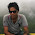3.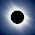You can declare 2 dimensional array where each sub array is of different length because its not mandatory to specify length of second dimension while declaring 2D array in Java. This way you can initialize 2D array with different length sub array as shown below :

String[][] squares = new String[];

squares = new String;
squares = new String;
squares = new String;

You can see that our 2 dimensional array contains 3 sub array each with different length 10, 20 and 30.

2.Is it possible to create hetrogenous two dimensional array in Java? Since 2D array is array of array, can I have array containing String[], Integer [] and Object[] as sub array?

1.Yes, its possible but only if you declare your 2D array as Object[][], which is array of Object array, as shown below :

Object[][] squares = new String[];

squares = new Integer[]{1, 3, 4,};
squares = new String[]{"A", "b"};
squares = new Float[]{1.0f, 2.0f};

You can see that our Object[][] is heterogeneous, it contains String[], Integer[] and Float[] all in one Object[].

2.so how can we get data? square = 3?

3.so how can we get an element? square = 3?

4.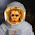so how we can get all above data like string,integer,and float??

3.how can we understand the flow of  matrices by taking for loop.could you please explain the flow

1.Hello Sameera, Similar to above example you can write nested loop, first will go through each row and second will go through each column. This way you will access all elements of matrix e.g.  first row first column, [0[ first row second column and  first row third column.

4.Well Explained

5.How to code this using two dimensional array in java? This is the output.
Enter 9 values:
1
2
3
4
5
6
7
8
9
1 2 3
4 5 6
7 8 9
Total: 45

1.import java.util.Scanner;

public class JunesBautista
{
public static void main(String args[])
{
int k=0;
int row, col, i, j;
int arr[][] = new int;
Scanner scan = new Scanner(System.in);

System.out.print("Enter Number of Row for Array (max 10) : ");
row = scan.nextInt();
System.out.print("Enter Number of Column for Array (max 10) : ");
col = scan.nextInt();

System.out.print("Enter " +(row*col)+ " Values : \n");
for(i=0; i<row; i++)
{
for(j=0; j<col; j++)
{
arr[i][j] = scan.nextInt();
k=k+arr[i][j];
}
}

for(i=0; i<row; i++)
{
for(j=0; j<col; j++)
{
System.out.print(arr[i][j]+ " ");
}
System.out.println();

}

System.out.println("Total is:" +k);

}

}

2.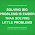1 2 3
8 9 4
7 6 5
i wont print like that plz help me

6.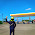ellow
can you code this..

this is the output

Please enter the grade of student 1
Q1;81
Q2;91
Q3;91
Please enter the grade of student 2
Q1;98
Q2;90
Q3;98

"up to 10 student"

Q1 Q2 Q3
Student 1 81 91 91
Student 98 90 98

this code are using Scanner and multi dimensional array
please help me..
thanks

7.char[] arr = ['0','1','~','n','a','m','e','1','~','h','y','d','&','0','2','~','n','a','m','e','2','~','p','u','n','e'];

It has sets of information like id, name, place seperated by a special char. Each set is again seperated by another special char.
Iterate over the arrays and display the information in the following format. The solution to this problems should be generic.

id : 01
name : name1
place : hyd

id : 02
name : name2
place : pune

Note : Do not use any JAVA API to get the output. Use only loops and conditional statements.

1.same with my case

8.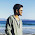how can i use 2d array in writing a code for login & registration details using only basic java knowledge?

9.can you codes this problem sir ? :)
Problem A: Single Dimensional Array
Prompt the user to enter array length. The Program will now store Double typed numbers and will get the average.

SAMPLE RUN:
Enter array size: 10
Enter 10 double – typed numbers:
80 99 85 100 77 78 96 100 85 100

The Average of stored numbers is 90.0
Problem B: Two-Dimensional Array
Ask your user to enter table dimensions. The user will save characters according to the size of the table. Display table items and create search item query, asking your user to enter table address.

SAMPLE RUN:
Enter number of rows: 3
Enter number of columns: 2
Enter 6 characters:
A J K L D O

Table items are:
A J
K L
D O

Enter Items to search: L
L is at row 1 column 1
Problem C: Class Vector
Sample Run:

Welcome to My Members List
[ 1 ] Add New Names
[ 2 ] Remove Names
[ 3 ] View Names
[ 4 ] Exit

Select Transaction: 1
Enter number of items to add: 3
Enter 3 names separated by space: Jessie Justin Darius
Items added! Your updated list are (Jessie, Justin, Darius)

Select Transaction: 2
Enter index number of item to remove: 1
Are you sure you want to remove this item? [Y / N]: Y
Item removed successfully. Your updated list are (Jessie, Darius)

Select Transaction: 3
Your String list are as follows:
(Jessie, Darius)

10.11.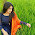how to print matrix representation in a webpage using jsp

12.import java.util.Arrays;
import java.util.Scanner;
public class MatrixDiagnal
{
public static void main (String args[])
{
int i,j,sum1=0,sum2=0,sum3=0,sum4=0,sum5=0;
int x[][] = new int ;
System.out.println("Type the digit:");
Scanner ob=new Scanner(System.in);
for(i=1;i<=3;++i)
{
for(j=1;j<=3;++j)
{
x[i][j]=ob.nextInt();
}
}
for(i=1;i<=3;++i)
{
for(j=1;j<=3;++j)
{
if(i==j)
{
sum1+=x[i][j];
}
if(i+j==2)
{
sum2+=x[i][j];
}
if(i!=1&&j!=1)
{
sum3+=x[i][j];
}
if(i!=1||j!=1)
{
sum4+=x[i][j];
}
if((j-i==1)||(j-i==0))
{
sum5+=x[i][j];
}
}
}
System.out.println(sum1);
System.out.println(sum2);
System.out.println(sum3);
System.out.println(sum4);
System.out.println(sum5);
}
}

13.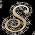1 2 3
6 5 4
7 8 9
i wont print like that please healp me

1.//ary is the 2d array, indents are messed up
for (int i = 0; i < ary.length; i++) {
for (int j = 0; j < ary[i].length; j++)
System.out.print(ary[i][j] + " ");
System.out.println();
}

2.int[][] a=new int;
int count=1;
for(int i=0;i<a.length;i++){
for(int j=0;j<a[i].length;j++){
a[i][j]=count++;
if(i==1 && j==0){
a[i][j]=6;
}else if(i==1 && j==2){
a[i][j]=4;
}

}
}
for(int[] in:a){
for(int ia: in){
System.out.print(ia+" ");
}
System.out.println("\n");
}

14.Branch1 Branch2 Branch3 Branch4 Branch5 Total Average(per Parts)
625 226 354 158 844 2207 441.4
225 154 251 331 154 1145 229
224 355 100 84 225 988 197.6
331 25 62 544 654 1616 469.2
588 664 354 555 185 2346 469.2

How is this guys?

15.How to initialize the array of type
int rows=3;
int s[] = new int[rows][];

Now how to initialize it?

16.How to initialize a global array in which number of columns varies?

eg:
int a[][] = new int[];

How to initialize it if I don't know the number of columns at present?

1.Hello @Akshay, you can do this with two dimensional array where just first index is mandatory but with one dimensional array you must specify number of items. You can see my post 6 ways to declare two dimensional array in Java for more details.

17.How do you fill a 2-D Array?

1.Hello @Anonymous, you can do this by using a 2 dimensional for loop, first loop will fill the rows and second will fill the columns.

18.Coordinate is (4,5) s = 6 I want to print like that:
https://s3.amazonaws.com/hr-assets/0/1502741023-54260789f9-Capture.PNG

19.Help please
The function takes two parameters: width
and height and returns with value populate in decreasing order. Consider the
following example:
width 4 and height 2
result would be

[8 7 6 5],
[4 3 2 1],